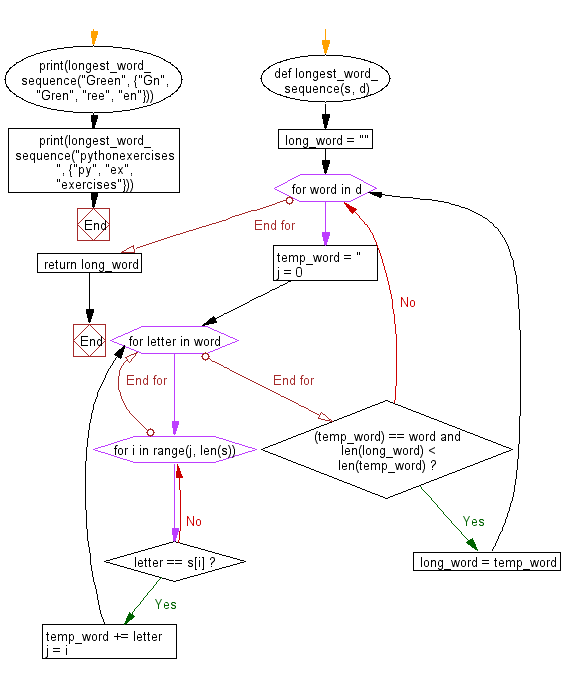﻿ Python: Find the longest word in set of words which is a subsequence of a given string - w3resource# Python: Find the longest word in set of words which is a subsequence of a given string

## Python Basic - 1: Exercise-65 with Solution

In mathematics, a subsequence is a sequence that can be derived from another sequence by deleting some or no elements without changing the order of the remaining elements. For example, the sequence (A,B,D) is a subsequence of (A,B,C,D,E,F) obtained after removal of elements C, E, and F. The relation of one sequence being the subsequence of another is a preorder.
The subsequence should not be confused with substring (A,B,C,D) which can be derived from the above string (A,B,C,D,E,F) by deleting substring (E,F). The substring is a refinement of the subsequence.
The list of all subsequences for the word "apple" would be "a", "ap", "al", "ae", "app", "apl", "ape", "ale", "appl", "appe", "aple", "apple", "p", "pp", "pl", "pe", "ppl", "ppe", "ple", "pple", "l", "le", "e", "".
Write a Python program to find the longest word in set of words which is a subsequence of a given string.

Sample Solution:

Python Code:

``````def longest_word_sequence(s, d):
long_word = ""

for word in d:
temp_word = ''
j = 0
for letter in word:

for i in range(j, len(s)):

if letter == s[i]:
temp_word += letter
j = i
break
else:
continue

if (temp_word) == word and len(long_word) < len(temp_word):
long_word = temp_word

else:
continue
return long_word

print(longest_word_sequence("Green", {"Gn", "Gren", "ree", "en"}))
print(longest_word_sequence("pythonexercises", {"py", "ex", "exercises"}))
``````

Sample Output:

```Gren
exercises
```

Pictorial Presentation:Flowchart:Python Code Editor:

Have another way to solve this solution? Contribute your code (and comments) through Disqus.

What is the difficulty level of this exercise?

Test your Programming skills with w3resource's quiz.

﻿

## Python: Tips of the Day

What is the difference between Python's list methods append and extend?

append: Appends object at the end.

```x = [1, 2, 3]
x.append([4, 5])
print (x)
```

Output:

```[1, 2, 3, [4, 5]]
```

extend: Extends list by appending elements from the iterable.

```x = [1, 2, 3]
x.extend([4, 5])
print (x)
```

Output:

```[1, 2, 3, 4, 5]
```

Ref: https://bit.ly/2AZ6ZFq